Miscellaneous

Chapter 2 Class 12 Inverse Trigonometric Functions
Serial order wise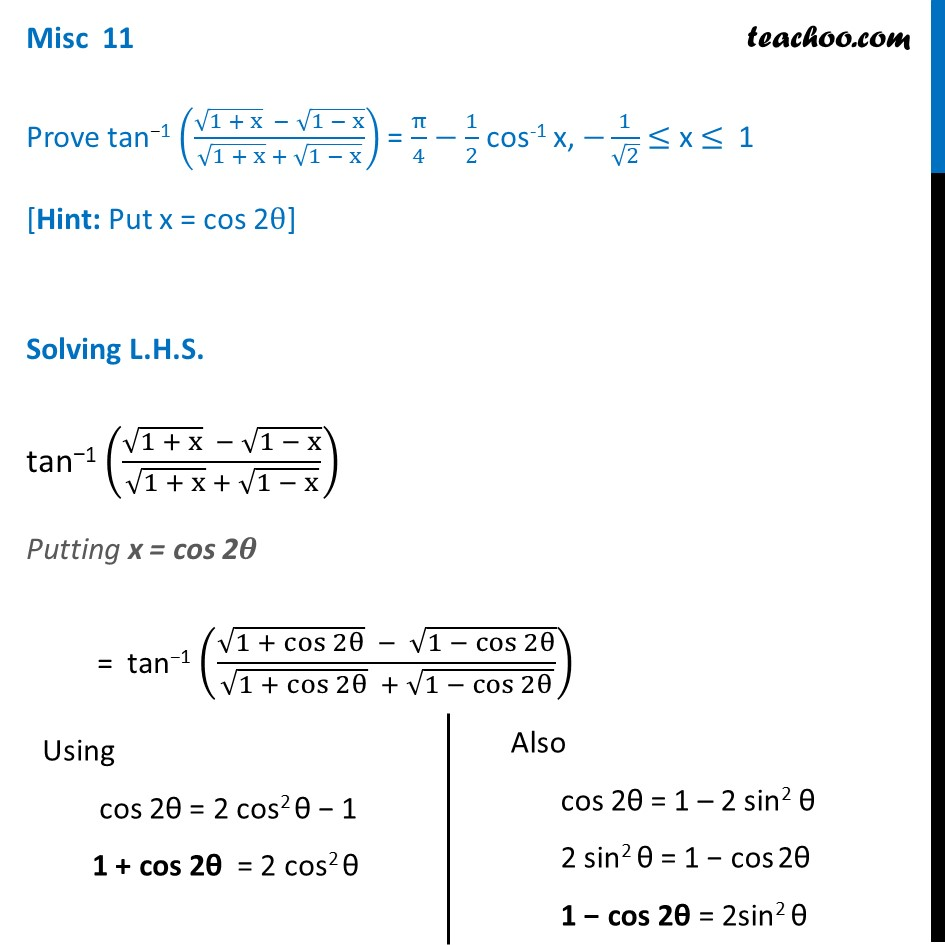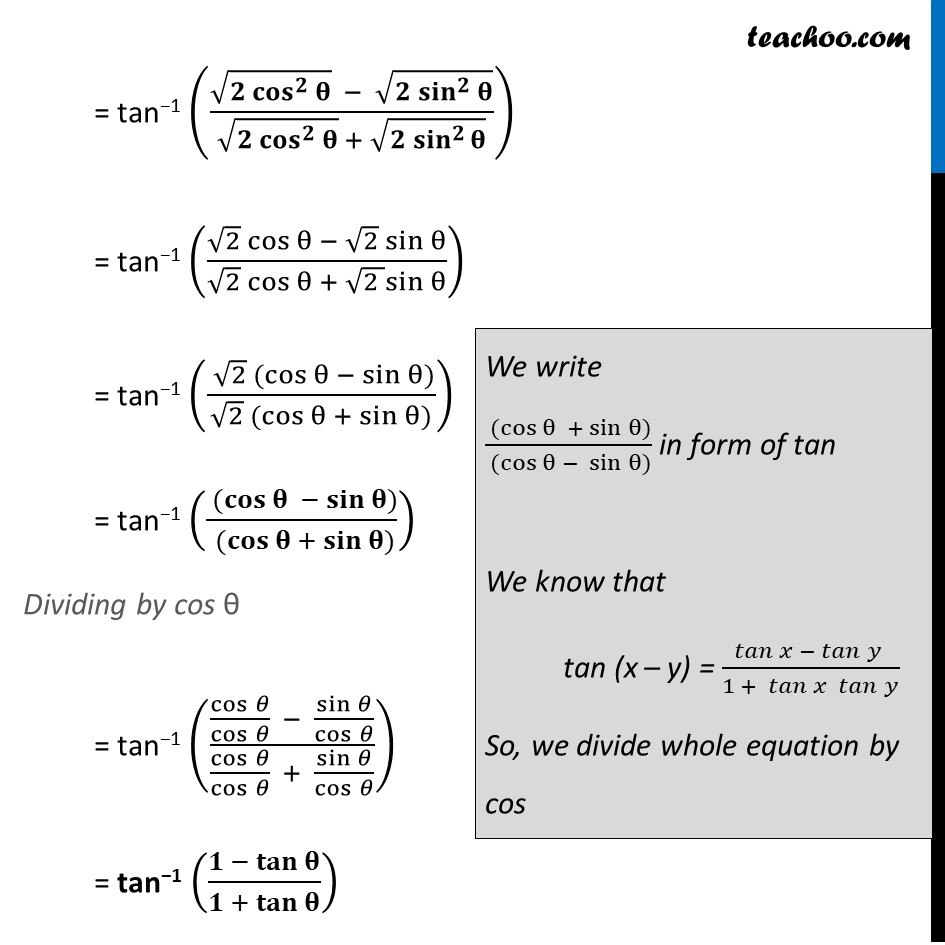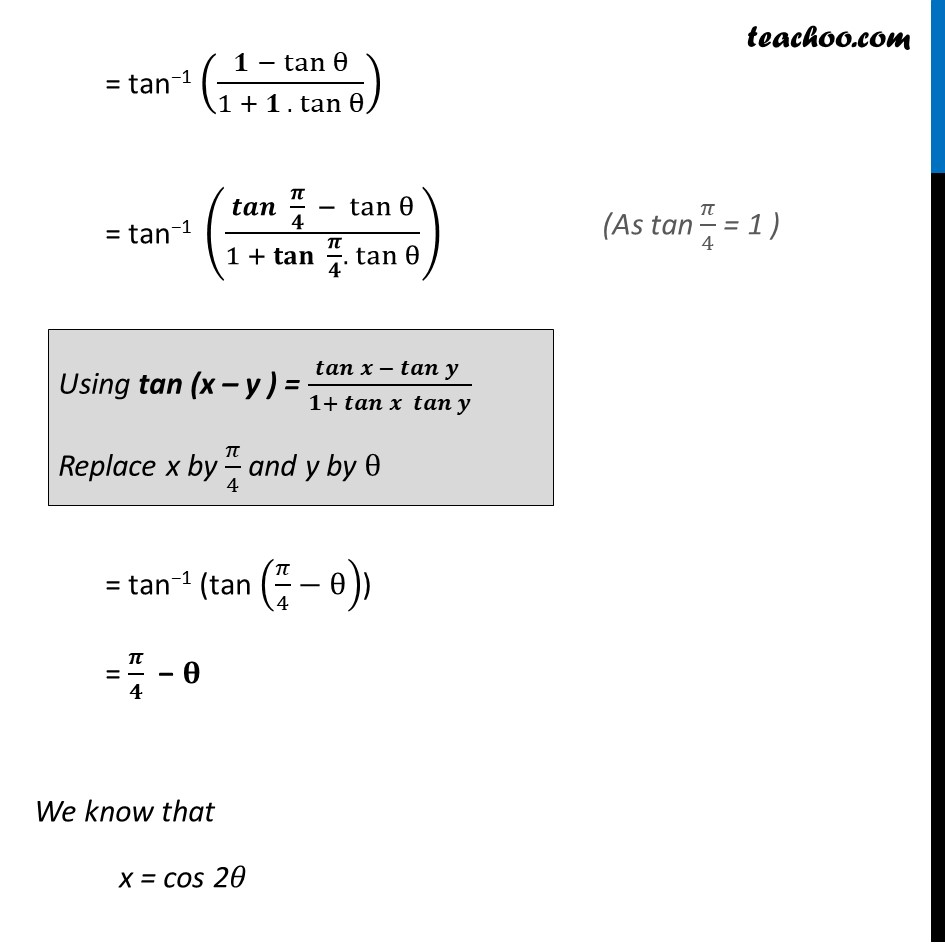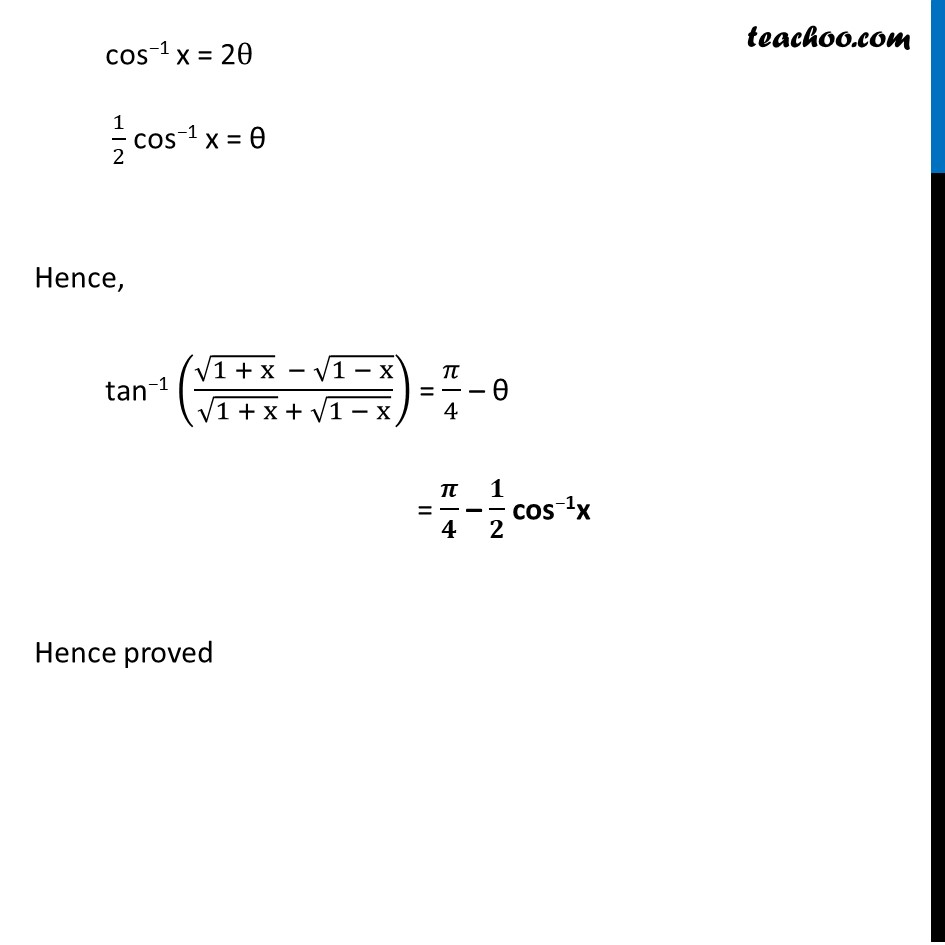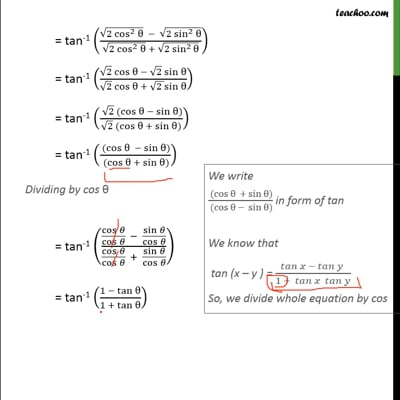This video is only available for Teachoo black users

Get live Maths 1-on-1 Classs - Class 6 to 12

### Transcript

Misc 11 Prove tan−1 ((√(1 + x) − √(1 − x))/(√(1 + x) + √(1 − x))) = π/4 − 1/2 cos-1 x, −1/√2 ≤ x ≤ 1 [Hint: Put x = cos 2θ] Solving L.H.S. tan−1 ((√(1 + x) − √(1 − x))/(√(1 + x) + √(1 − x))) Putting x = cos 2𝜽 = tan−1 ((√(1 + cos 2θ) − √(1 − cos 2θ))/(√(1 + cos 2θ) + √(1 − cos 2θ)))Using cos 2θ = 2 cos2 θ − 1 1 + cos 2θ = 2 cos2 θ Also cos 2θ = 1 – 2 sin2 θ 2 sin2 θ = 1 − cos 2θ 1 − cos 2θ = 2sin2 θ = tan−1 ((√(𝟐 〖𝐜𝐨𝐬〗^𝟐 𝛉) − √(𝟐 〖𝐬𝐢𝐧〗^𝟐 𝛉))/(√(𝟐 〖𝐜𝐨𝐬〗^𝟐 𝛉) + √(𝟐 〖𝐬𝐢𝐧〗^𝟐 𝛉))) = tan−1 ((√2 cos θ − √2 sin θ)/(√2 cos θ + √(2 ) sin θ)) = tan−1 (( √2 (cos θ − sin θ))/(√2 (cos θ + sin θ))) = tan−1 (( (𝐜𝐨𝐬 𝛉 − 𝐬𝐢𝐧 𝛉))/( (𝐜𝐨𝐬 𝛉 + 𝐬𝐢𝐧 𝛉))) Dividing by cos θ = tan−1 ((cos⁡𝜃/cos⁡𝜃 − sin⁡𝜃/cos⁡𝜃 )/(cos⁡𝜃/cos⁡𝜃 + sin⁡𝜃/cos⁡𝜃 )) = tan−1 ((𝟏 − 𝐭𝐚𝐧 𝛉)/(𝟏 + 𝐭𝐚𝐧 𝛉)) We write ( (cos θ + sin θ))/( (cos θ − sin θ)) in form of tan We know that tan (x – y) = 𝑡𝑎𝑛⁡〖𝑥 −〖 𝑡𝑎𝑛〗⁡〖𝑦 〗 〗/(1 + 𝑡𝑎𝑛⁡〖𝑥 𝑡𝑎𝑛⁡𝑦 〗 ) So, we divide whole equation by cos = tan−1 ((𝟏 − tan θ)/(1 + 𝟏 . tan θ)) = tan−1 ((𝒕𝒂𝒏⁡〖 𝝅/𝟒〗 − tan⁡θ)/(1 + 𝐭𝐚𝐧⁡〖 𝝅/𝟒〗.〖 tan〗⁡θ )) = tan−1 (tan (𝜋/4−θ)) = 𝝅/𝟒 − 𝛉 We know that x = cos 2𝜃 Using tan (x – y ) = 𝒕𝒂𝒏⁡〖𝒙 −〖 𝒕𝒂𝒏〗⁡〖𝒚 〗 〗/(𝟏+ 𝒕𝒂𝒏⁡〖𝒙 𝒕𝒂𝒏⁡𝒚 〗 ) Replace x by 𝜋/4 and y by θ (As tan 𝜋/4 = 1 ) cos−1 x = 2θ 1/2 cos−1 x = θ Hence, tan−1 ((√(1 + x) − √(1 − x))/(√(1 + x) + √(1 − x))) = 𝜋/4 – θ = 𝝅/𝟒 – 𝟏/𝟐 cos−1x Hence proved